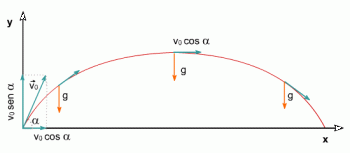# Projectile Motion - Formulas, Examples and Solved ExerciseProjectile motion - or parabolic motion - is a type of motion in which an object is thrown with an initial velocity at a given angle to the ground and then follows a parabolic trajectory due to the action of gravity.

The projectile motion can be defined as the sum of two motions:

• The horizontal component corresponds to the uniform rectilinear motion.

• The vertical component corresponds to the uniformly accelerated rectilinear movement in which the acceleration is a consequence of gravity.

This movement is very common in everyday life and has important applications in various areas such as physics, engineering, and aeronautics, among others.

## Parabolic Motion Formulas

The formulas for projectile motion in kinematics are:

### Position EquationsWhere:

• x0 , y0 are the initial coordinates

• v0 is the initial velocity

• α is the angle that the velocity vector makes with the horizontal

• g is the acceleration due to gravity (-9.8 m/s 2 on Earth)

• t is the elapsed time

### Velocity EquationsWhere:

• vfx , vfy are the horizontal and vertical components of the final velocity.
• v0 is the initial velocity.
• α is the angle that the velocity vector makes with the horizontal.
• g is the acceleration due to gravity (-9.8 m/s2 on Earth).
• t is the elapsed time.

## The Components of Velocity

In most calculations the initial velocity and the angle it makes with the horizontal are known. In any case, the velocity can be broken down into its horizontal and vertical components using trigonometry:

• Horizontal component: v x =v cos(α)

• Vertical component: v y = v sin(α)

In the same way, when we have the speed given in its two components, we can know the total speed using the Pythagorean theorem:## Projectile Motion Applications

The applications of the parabolic shot are very diverse. For example, in the sports field, the throwing of balls in sports such as soccer, basketball, and baseball, among others, are governed by the laws of parabolic shooting.

In engineering, analyzing parabolic movement is essential in designing bridges, viaducts, and any structure that must support loads moving in this trajectory.

In physics, the parabolic shot is used to analyze the motion of projectiles and to study the kinematics and dynamics of moving objects.

## How Does Gravity Affect It?

Gravity is the determining factor in projectile motion as it is the constant force acting on the object. Acceleration due to gravity affects the object by pulling it toward the center of the Earth, producing a constant downward acceleration. This makes the trajectory of the object curved and follows a parabolic shape.

Gravity also influences the height and range of the thrown object. The maximum height the object reaches depends on the initial velocity, launch angle, and the gravitational force pulling the object downwards.

## Projectile Motion Examples

There are many examples of projectile motion in everyday life. Below are some of them:

1. A basketball player shooting a basketball toward the basket: When a player throws it toward the basket, it follows a parabolic trajectory due to gravity. The ball is thrown with an initial velocity and a certain angle and falls towards the basket in a parabolic trajectory after reaching its maximum height.

2. A baseball thrown by a pitcher: When a pitcher throws a baseball toward the catcher, it follows a parabolic trajectory. The ball is thrown with an initial velocity and a certain angle, and after reaching its maximum height, it falls towards the receiver in a parabolic trajectory.

3. An object thrown from a building or platform: When thrown from a building or platform, it follows a parabolic path due to gravity. The object is launched with an initial velocity and a certain angle, and after reaching its maximum height, it falls toward the ground in a parabolic trajectory.

4. A plane dropping a bomb: When it drops a bomb, it follows a parabolic trajectory due to gravity. The bomb is thrown with an initial velocity and a certain angle, and after reaching its maximum height, it falls towards the target in a parabolic trajectory.

5. A ski jumper jumping off a springboard: When a ski jumper jumps off a springboard, they follow a parabolic path through the air before landing on the slope. The jumper is launched with an initial velocity and a certain angle, and after reaching its maximum height, it falls towards the slope in a parabolic trajectory.

## Solved Exercise

Suppose we throw a ball from a height of 2 meters above the ground, with an initial velocity of 10 m/s and a launch angle of 45 degrees. What is the maximum height the ball reaches, and how long does it take to return to the ground?

We know that at the highest point, the vertical component of velocity is zero. So then, using the formula for speed, we can see the time it takes:Now, with the vertical position formula, we calculate the final position after t seconds:Therefore, the ball's maximum height is 1.5435 meters above the ground.

Author:

Published: May 9, 2023
Last review: May 9, 2023